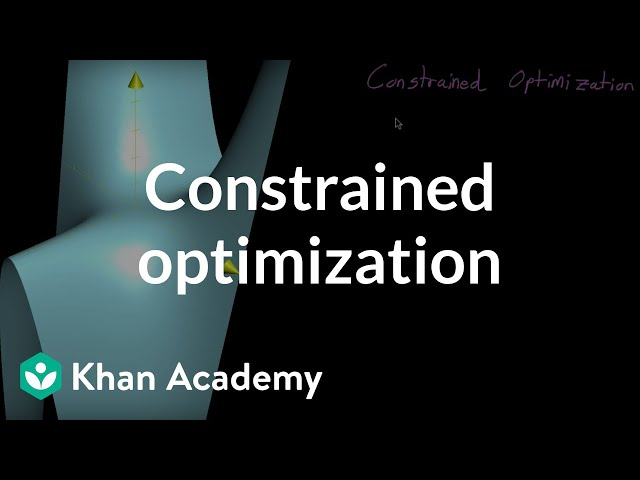# What is Constrained Optimization

##### Constrained Optimization: Achieving Optimal Solutions with Constraints

Optimization problems are prevalent in numerous areas of science and engineering, ranging from finance to physics, and from computer science to economics. Although straightforward optimization problems can be solved by finding the optimal solution that maximizes or minimizes a given objective function, real-world situations often involve more complex constraints, such as physical or operational limitations, budget constraints, or legal requirements. In such cases, constrained optimization techniques are required to find the optimal solution that satisfies all the constraints. This article provides an overview of constrained optimization, its applications, and some of the most commonly used methods.

##### The Basics of Constrained Optimization

Constrained optimization refers to the process of finding the optimal solution to an optimization problem while satisfying certain constraints. It involves minimizing or maximizing a given objective function subject to a set of constraints. These constraints limit the feasible region of the optimization problem, ensuring that the solution found is both optimal and feasible. For instance, an optimization problem that involves maximizing profits subject to a certain production capacity constraint can be formulated as:

Maximize profits = revenue - costs

Subject to the constraint: production capacity ≤ maximum capacity

In this example, the objective function is to maximize profits, while the constraint limits the feasible region to production levels that do not exceed the maximum capacity. Such constraints can be expressed as equalities or inequalities, depending on the problem's formulation. Constrained optimization problems can be solved using a range of methods, including linear programming, quadratic programming, and semidefinite programming. These methods apply different algorithms to solve problems with different levels of complexity and constraint types.

##### Applications of Constrained Optimization

Constrained optimization is widely used in many fields, including engineering, economics, finance, energy, transportation, and logistics. Some examples of optimization problems with constraints are:

• Maximizing the profit of a company subject to resource constraints
• Minimizing the energy consumption of a building subject to temperature and humidity restrictions
• Optimizing the placement of wind turbines to maximize energy production while considering land use and environmental constraints
• Minimizing the travel time of a delivery truck while considering load constraints and road conditions
• Optimizing the production schedule of a manufacturing plant while considering labor and raw material constraints

In each of these examples, the constrained optimization problem has a specific objective, but the solution must satisfy certain operational, economic, or environmental constraints. Constrained optimization methods are essential to find the optimal solutions to these problems while ensuring that the constraints are met.

##### Commonly Used Constrained Optimization Methods

Several methods are available for solving constrained optimization problems. The choice of method depends on various factors such as the problem's complexity, the type of constraints, and the size of the problem. Here is a brief overview of some commonly used constrained optimization methods:

• Linear programming: Linear programming (LP) is a method for solving optimization problems that involve linear constraints and a linear objective function. LP optimizes problems with a small number of variables and constraints, making it faster and more efficient than other methods for this type of problem. LP problems can be solved using simplex algorithms or interior-point methods.
• Quadratic programming: Quadratic programming (QP) is a method for solving optimization problems that involve quadratic terms in both the objective function and the constraints. QP is useful for problems with a small to medium number of variables and constraints. The most commonly used methods are active set algorithms and interior-point methods.
• Nonlinear programming: Nonlinear programming (NLP) is a method for solving optimization problems that involve nonlinear constraints or objective functions. NLP has various applications in engineering, design, and manufacturing. The most commonly used methods are sequential quadratic programming, interior-point methods, and genetic algorithms.
• Dynamic programming: Dynamic programming (DP) is a method for solving optimization problems that involve a sequence of decisions over time. DP takes into account the relationship between the current decision and future outcomes, making it useful for problems that require long-term planning. DP can be applied to a range of problems such as inventory management, production schedules, and financial planning.
• Integer programming: Integer programming (IP) is a method for solving optimization problems in which the decision variables must be integers. IP has numerous applications in staffing, scheduling, and routing problems. The most commonly used methods are branch-and-bound and cutting-plane algorithms.

Other methods such as stochastic programming, global optimization, and constraint satisfaction are also used for solving specific types of constrained optimization problems.

##### Conclusion

Constrained optimization is an essential tool for solving complex optimization problems with constraints. It allows practitioners to find the optimal solutions that satisfy all operational, economic, and environmental constraints, while avoiding infeasible or unworkable solutions. The choice of method depends on various factors such as the complexity of the problem, the type of constraints, and the size of the problem. The most commonly used methods include linear programming, quadratic programming, nonlinear programming, dynamic programming, and integer programming. As optimization problems become increasingly complex and real-world applications more diverse, constrained optimization techniques will continue to be critical for finding optimal solutions.